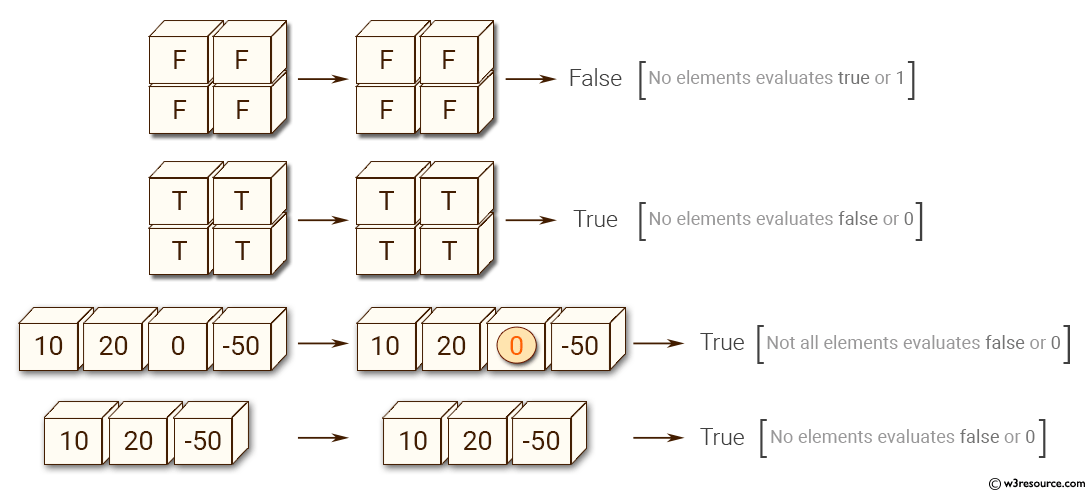﻿ NumPy: Test whether any array element along a given axis evaluates to True - w3resource# NumPy: Test whether any array element along a given axis evaluates to True

## NumPy: Array Object Exercise-24 with Solution

Write a NumPy program to test whether any array element along a given axis evaluates to True.
Note: 0 evaluates to False in python.

Pictorial Presentation:Sample Solution:-

Python Code:

``````import numpy as np
print(np.any([[False,False],[False,False]]))
print(np.any([[True,True],[True,True]]))
print(np.any([10, 20, 0, -50]))
print(np.any([10, 20, -50]))
```
```

Sample Output:

```False
True
True
True
```

Python Code Editor:

Have another way to solve this solution? Contribute your code (and comments) through Disqus.

What is the difficulty level of this exercise?

Test your Python skills with w3resource's quiz

﻿

## Python: Tips of the Day

Set comprehension:

```>>> m = {x ** 2 for x in range(5)}
>>> m
{0, 1, 4, 9, 16}
```